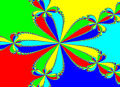# 97松山家商

## 97松山家商

111.7.12補充

$$\Delta=\left| \matrix{a_1&b_1&c_1\cr a_2&b_2&c_2\cr a_3&b_3&c_3}\right|=0$$，$$\Delta_x=\left| \matrix{d_1&b_1&c_1\cr d_2&b_2&c_2\cr d_3&b_3&c_3}\right|$$、$$\Delta_y=\left| \matrix{a_1&d_1&c_1\cr a_2&d_2&c_2\cr a_3&d_3&c_3}\right|$$、$$\Delta_z=\left| \matrix{a_1&b_1&d_1\cr a_2&b_2&d_2\cr a_3&b_3&d_3}\right|$$，

#### 附件97松山家商.pdf (82.31 KB)

2012-1-1 00:19, 下載次數: 815097松山家商答案.pdf (42.6 KB)

2012-1-1 00:19, 下載次數: 7748

TOP

 weiye 瑋岳發私訊 加為好友 目前離線 2# 大 中 小 發表於 2008-12-3 18:28  只看該作者 填充題，第四題： 我的做法： 令 f（x,y）＝ （x＋y － |x－y|）／2 ， 依照定義 　　若 x≧ y 則 f（x,y）＝y 　　若 x＜y 則 f（x,y）＝x 　　亦即，f（x,y） 就是求 x,y 兩數中的最小值 當數對 （x,y） 的最小值是 k 時，情形有 （k, k）, （k,k＋1）, ... （k,6） 及 （k＋1,k）, ..., （6,k） 共 2×（7－k）－1 種 所以期望值＝ 1×（2×6－1）／36 ＋ 2×（2×5－1）／36 ＋ ... ＋ 2×（2×1－1）／36 ＝ 中間過程用 Σ 算一下 ＝ 91／36. 計算題，第二題： 我的做法： Ｃ（n,r）：Ｃ（n,r＋1）：Ｃ（n,r＋2） ＝ 1：m：2m 把前兩個與後兩個比例分開來寫，可得 mＣ（n,r） ＝ Ｃ（n, r＋1） 且 2Ｃ（n, r＋1） ＝ Ｃ（n, r＋2） ⇒　m×n！／（r！（n－r）！） ＝ n！／（（r＋1）！（n－r－1）！） 　　且 2×n！／（（r＋1）！（n－r－1）！） ＝ n！／（（r＋2）！（n－r－2）！） 把可以約分的約掉，並且同乘兩邊的分母，可得 ⇒ 　m（r＋1）＝（n－r） .....（＊）　且 2（r＋2） ＝ （n－r－1）......（＊＊） 將（＊）中的 n－r 帶入 （＊＊）中，可得 　　2（r＋2） ＝ m（r＋1）－1.....（＊＊＊） ⇒ 　2r＋5 ＝ m（r＋1） ⇒ 　r＋1 ｜ 2r＋5 且因為 r＋1 ｜ r＋1 所以　r＋1 ｜ （2r＋5）－2（r＋1） ⇒ 　r＋1 ｜ 3 ⇒ 　r＋1 ＝ 1 or 3 ⇒ 　r＝0 or 2 帶回 （＊＊）可得 n 值 ，帶回 （＊＊＊） 可得 m 值， 故，有序數組 （m,n,r） ＝ （5,5,0） 或 （3,11,2）. 計算題，第三題： 我的做法： 設通過 S1 與 S2 交圓的所求球面方程式為 　　（x－2）^2 ＋ （y－2）^2 ＋ （z－2）^2 －9 ＋ k{ x^2 ＋ y^2 ＋ z^2 － 1} ＝ 0 ..... （＊） 將 y＝0, z＝0 帶入（＊），找出所求球面與 x 軸的交點坐標的 x 坐標為兩根的方程式， 　　（1＋k）x^2 － 4x ＋ （3－k） ＝0 利用根與係數關係式，可得  兩根之和＝ 4／（1＋k），兩根之積＝（3－k）／（1＋k） 依題意，可知兩根之差的絕對值＝4， 利用　（α－β）^2 ＝ （α＋β）^2 － 4αβ 可得　4^2 ＝ （4／（1＋k））^2 － 4（（3－k）／（1＋k）） 解 k 的一元二次方程式，得 k ＝ －3 或 －1／3， 帶回 （＊） ，即可得答案. UID1 帖子2182 閱讀權限200 上線時間8161 小時 註冊時間2006-3-5 最後登入2023-5-29  查看詳細資料 TOP

## 回復 2# weiye 的帖子

TOP

 tsusy 寸絲發私訊 加為好友 目前離線 4# 大 中 小 發表於 2014-8-24 16:13  只看該作者 計算證明4 $$\Delta ABC$$中，設$$a,b,c$$分別為其三內角$$∠A,∠B,∠C$$的對邊，$$\Delta$$為其面積，而$$R$$為其外接圓半徑 (1)試證：$$\displaystyle cot A=\frac{b^2+c^2-a^2}{4 \Delta}$$ (2)利用(1)證明：若$$cot A,cot B,cot C$$成等差數列，則$$a^2,b^2,c^2$$亦成等差數列 (3)如圖：設$$\Delta ABC$$內部一點$$P$$，使得$$∠PAB=∠PBC=∠PCA=\alpha$$，利用(1)證明：$$cot \alpha=cot A+cot B+cot C$$ (4)試證：$$a^2+b^2+c^2 \ge 4 \sqrt{3} \cdot \Delta$$ 借標題向諸位請教，計算證明 4.(4) 怎麼用前面小題的結論會比較方便 在 4(3) 中，有先得到 $$a^2 + b^2 + c^2 = 4 \triangle \cot \alpha$$ 如果可以證出 $$\alpha \leq 30^\circ$$ 加上布洛卡兒點的存在性? 即可得證 (4) 先給個無關題組的另證1： 由算幾不等式可得 $$\frac{a^{2}+b^{2}+c^{2}}{3}\geq\sqrt{a^{2}b^{2}c^{2}} \Rightarrow\left(a^{2}+b^{2}+c^{2}\right)^{3}\geq27a^{2}b^{2}c^{2}$$ $$\frac{a}{2}=\frac{s-b+s-c}{2} \Rightarrow\frac{a}{2}\geq\sqrt{(s-b)(s-c)} \Rightarrow a^{2}\geq4(s-b)(s-c)$$ (a,b,c 可互換) 結合上兩行得 $$\left(a^{2}+b^{2}+c^{2}\right)^{3}\geq3^{3}a^{2}b^{2}c^{2}\geq12^{3}(s-a)^{2}(s-b)^{2}(s-c)^{2}$$。 由柯西不等式有 $$(a^{2}+b^{2}+c^{2})\cdot(1+1+1)\geq(a+b+c)^{2} \Rightarrow a^{2}+b^{2}+c^{2}\geq\frac{4}{3}s^{2}$$。 結合上兩行得 $$\left(a^{2}+b^{2}+c^{2}\right)^{4}\geq\frac{12^{4}}{9}\triangle^{4} \Rightarrow a^{2}+b^{2}+c^{2}\geq4\sqrt{3}\triangle$$。 另證2： $$a^{2}+b^{2}+c^{2}=\frac{a^{2}+b^{2}+c^{2}+a^{2}+b^{2}+c^{2}}{2}\geq ab+bc+ca=2\triangle(\csc A+\csc B+\csc C)$$ 而 $$(\csc\theta)''=\frac{2\cos^{2}\theta}{\sin^{3}\theta}+\frac{1}{\sin\theta}$$，故 $$\csc\theta$$ 在 $$(0,\pi)$$ 上為凸函數 (凹向上)，因此 $$\frac{\csc A+\csc B+\csc C}{3}\geq\csc60^{\circ}=\frac{2}{\sqrt{3}}$$， 所以 $$a^{2}+b^{2}+c^{2}\geq=2\triangle(\csc A+\csc B+\csc C)\geq4\sqrt{3}\triangle$$。 網頁方程式編輯 imatheq UID981 帖子1057 閱讀權限10 來自方寸之地 上線時間2984 小時 註冊時間2011-10-10 最後登入2023-5-28  查看個人網站 查看詳細資料 TOP
##### 引用:

P 在 △ABC 內部，證明 ∠PAB、∠PBC、∠PCA 三者中至少有一個 ≦ 30∘

TOP

TOP

## 回復 6# tsusy 的帖子

a²+b²+c²-4√3*△
=(b²+c²-2bc*cosA)+b²+c²-4√3*(1/2)bc*sinA  (餘弦定理+面積公式)
=2{ b²+c²-2bc[cosA*(1/2) +sinA*(√3/2)] }
=2[ b²+c² -2bc*sin(A+30度) ]
>=2 (b²+c² -2bc)
=2(b-c)²
>=0

a²+b²+c²>=4√3*△+(a-b)²+(b-c)²+(c-a)²   (提升下界)

[ 本帖最後由 Ellipse 於 2014-8-24 10:25 PM 編輯 ]

TOP

 deca0206發私訊 加為好友 目前離線 8# 大 中 小 發表於 2015-8-23 11:02  只看該作者 想請教填充第二題，感謝 已知方程式$$x^5-32=0$$的四個相異虛根為$$\alpha,\beta,\gamma,\delta$$，設$$f(x)=x^3+x^2+1$$，則$$f(\alpha)+f(\beta)+f(\gamma)+f(\delta)=$$　　　。 UID1931 帖子34 閱讀權限10 上線時間18 小時 註冊時間2015-4-21 最後登入2019-5-16  查看詳細資料 TOP

## 回復 8# deca0206 的帖子

\begin{align} & \alpha =2\omega ,\beta =2{{\omega }^{2}},\gamma =2{{\omega }^{3}},\delta =2{{\omega }^{4}},\omega =\cos \frac{2\pi }{5}+i\sin \frac{2\pi }{5} \\ & {{\omega }^{5}}=1 \\ & {{\omega }^{4}}+{{\omega }^{3}}+{{\omega }^{2}}+\omega =-1 \\ & f\left( \alpha \right)+f\left( \beta \right)+f\left( \gamma \right)+f\left( \delta \right)={{2}^{3}}\left( {{\omega }^{3}}+\omega +{{\omega }^{4}}+{{\omega }^{2}} \right)+{{2}^{2}}\left( {{\omega }^{2}}+{{\omega }^{4}}+\omega +{{\omega }^{3}} \right)+4=-8 \\ \end{align}

[ 本帖最後由 thepiano 於 2015-8-23 12:11 PM 編輯 ]

TOP

 deca0206發私訊 加為好友 目前離線 10# 大 中 小 發表於 2015-8-23 12:32  只看該作者 謝謝老師 UID1931 帖子34 閱讀權限10 上線時間18 小時 註冊時間2015-4-21 最後登入2019-5-16  查看詳細資料 TOP
﻿